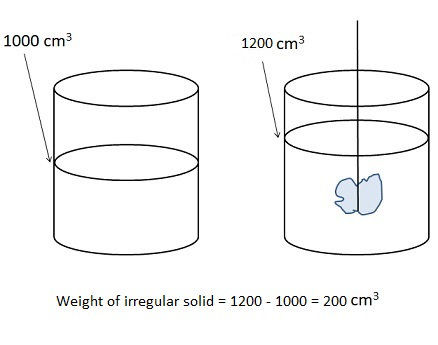## Measurement

Physical quantity: A quantity which can be measured is called physical quantity. E.g. length, mass, capacity, force, time, temperature, energy etc.

Standard unit: A unit which is used to measure a physical quantity and which remains same at all times and conditions is called standard unit.

S.I. (Systeme Internationale or International System): A standard unit which is accepted internationally for measurement of a physical quantity is known as its S.I. unit. E.g. S.I. unit of length is meter, S.I. unit of mass is kg etc.

 Physical Quantity S.I. Unit Length metre (m) Mass kilogram (kg) Capacity (Volume) litre (l) Time second (s) Temperature Kelvin (K) Force (Weight) Newton (N) Electric current Ampere (A) Pressure Pascal (Pa)

Important concepts:

• Mass can be measured in gram, ton, ounce, pound, stone and carat.
• Ounce and carat are used to measure very light objects.
• Length can be measured in inch, foot, yard, miles, millimeter, centimeter, meter, kilometer, lightyear, angstrom etc.
• Very small lengths line wavelengths, size of atoms, length of chemical bonds etc are measured in angstrom. 1 angstrom=10- 8m.
• Very large distance like the distance between earth and sun is measured in lightyears.
• 1 Lightyear is the distance travelled by light in a year in a perfect vacuum.
• The distance between 2 cities is measured in kilometers.
• Length of cloth is usually measured in meters.
• An odometer is an instrument in vehicles which is used for measuring distance.
• A speedometer is a gauge in vehicles which shows speed.
• Volume or capacity is measured in litre, cubic centimeters etc.
• To measure the volume of irregular solids they are immersed in measuring cylinders and the volume of liquid displaced by them is equal to their volume.